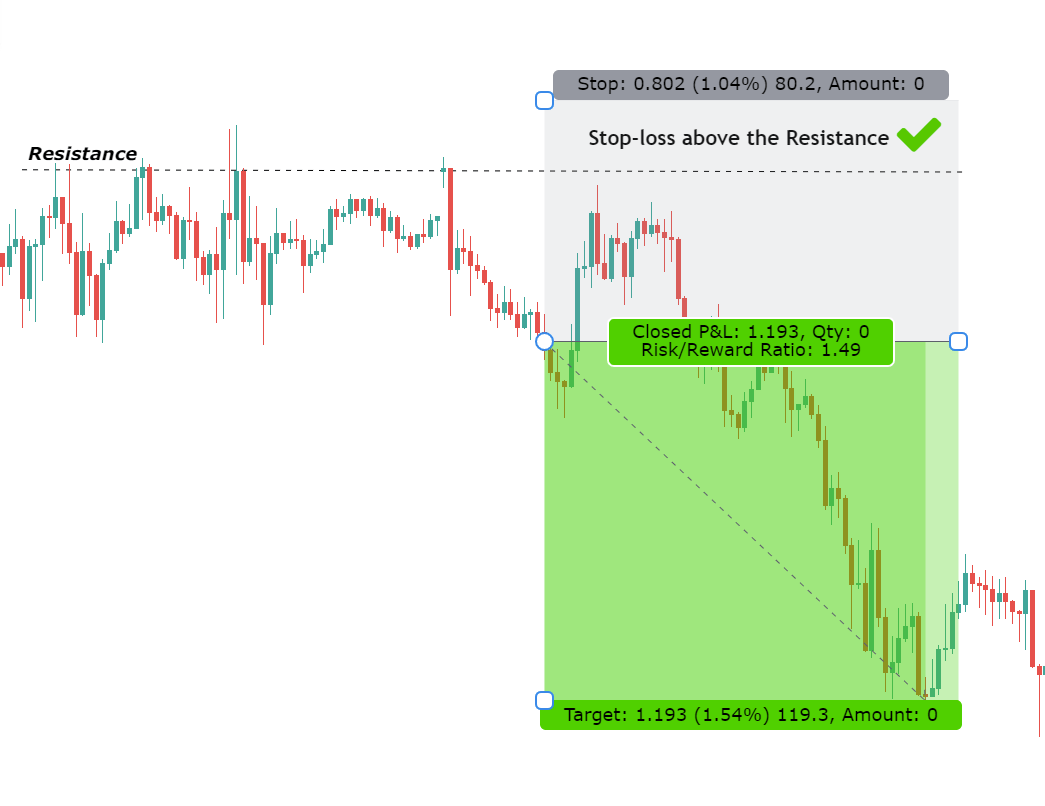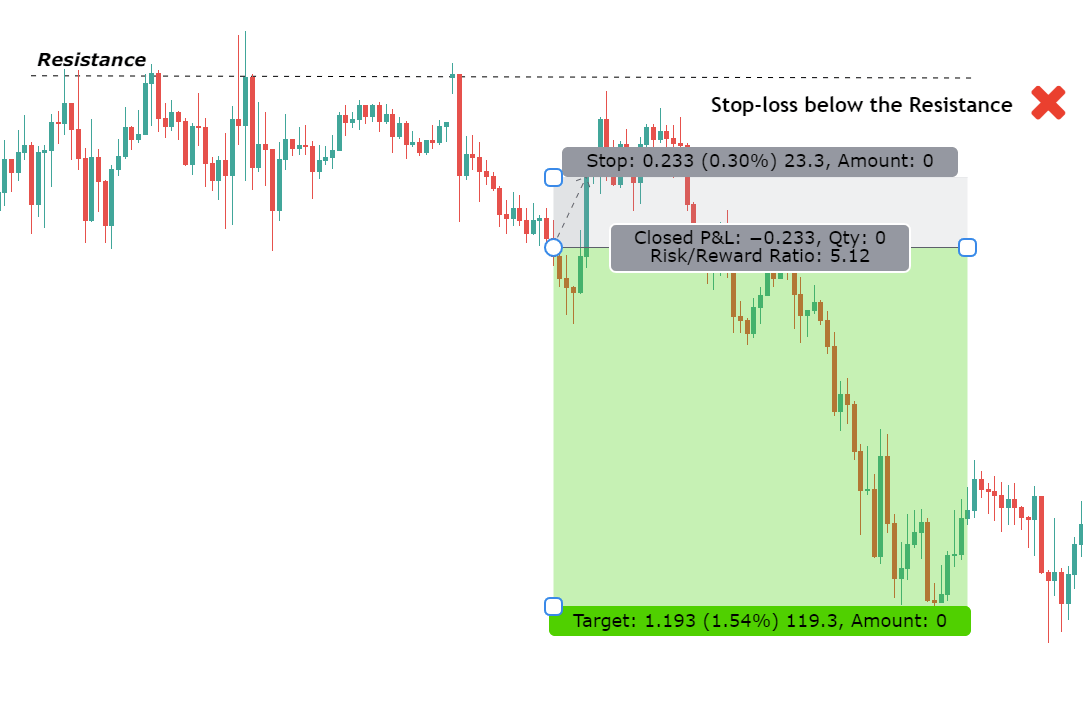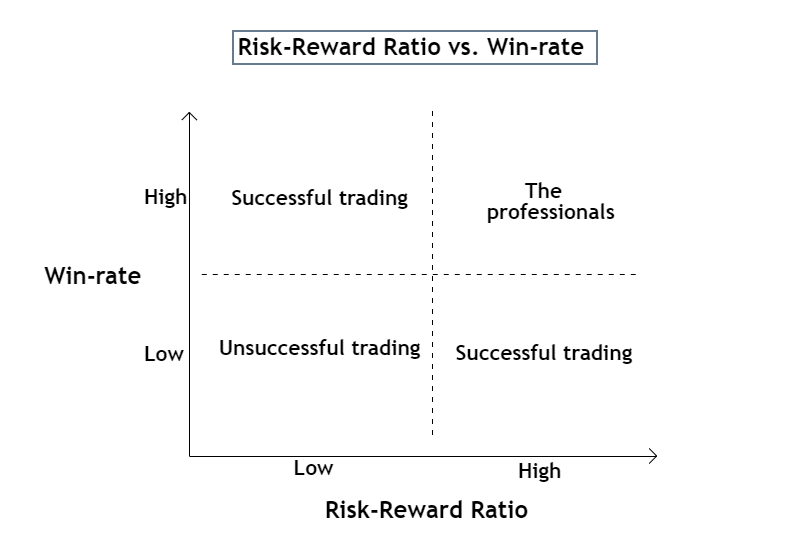# A Definitive Guide to Risk Reward Ratio in Forex Trading

This article will be going through the fine details of the "Risk-Reward Ratio in Forex Trading" and understand the critical relationship between risk-reward ratio & win-rate in a trading strategy.

## Introduction

As per the statistics, only 5% of all the traders become successful in trading. There are several reasons why most traders fail to find an edge in their trading. One of the common reasons behind the trader's failure is ignorance of money-management and risk-management.

Having a good trading strategy in place is vital but not sufficient. It is also crucial to managing risk smartly and efficiently.

There are traders whose win-rate percentage is not more than 50%, yet they are successful. This is possible because the applied risk-management is excellent and better than an average trader.

Precisely speaking, it is the risk-reward ratio that matters most in trading. If a trader understands the concept of risk-reward ratio in Forex trading, they can prosper even with an average winning rate.

## The Prerequisites

Before diving into understanding the risk-reward ratio, it is vital to comprehend some closely related terms.

### The Stop-Loss

Stop-loss is a limit order that is placed against the trader's desired direction, to cut losses. A stop-loss must be placed using a systematical approach.

Novice traders place their stop-loss at a price where they cannot endure more loss (in dollars or equivalent currency), and that's a wrong approach.

A stop-loss must be placed at a level where the price should react off from it. Besides, if the price does hit the stop-loss, it would indicate that the market has changed its structure and could continue in the other direction.

### Where to place the Stop-Loss?

The best place to put your stop-loss is around the Support and Resistance levels.

Many traders decide the stop-loss based on the risk-appetite. As a result, they end up getting stopped out the majority of the time.

Below is a self-explanatory example supporting the above statement.### The Take-Profit

Take-profit is also a limit order typically placed to close a trade position in a profit.

It is placed above the entry price in a long position and below the entry price in a short position. Similar to stop-loss, take-profit must be calculated and placed logically.

Contrary to popular opinion, they let their losses run and cut the profits. Due to this, they end up in negative even after having a high winning percentage.

Having a clear take-profit in advance will prevent any silly decisions during the trade.

The interpretation of the risk-reward ratio revolves around the stop-loss and take-profit.

Now that we understood these two terms, we can now better understand the risk-reward ratio in Forex trading.

## The Basics of Risk-Reward Ratio in Forex Trading

The risk-reward ratio, also known as R/R ratio, is a measure that compares the potential trade profit with loss and depicts as a ratio.

It assesses the reward (take-profit) of a trade by comparing the risk (stop loss) involved in it.

The risk-reward represents how many dollars one must risk to make a specified dollar amount. The relationship between the two values yields another value that determines if it is worth taking a trade or not.

## How is the Risk-Reward Ratio calculated?

To calculate the risk-reward ratio on a trade, the risk and rewards must be defined separately.

### Risk

Risk is a potential loss on the trade, represented by a stop-loss. Note that, we are interested in the amount that could be lost, and not the price where the stop-loss is placed.

Risk = Entry price ~ Stop-loss price

### Reward

The reward is the potential profit obtainable from the trade, using the take-profit. It represents the dollar amount that can be gained from trade.

Reward = Take-profit ~ Entry price

## The Formula of Risk-Reward Ratio

Risk-Reward Ratio = (Entry price ~ Stop-loss price) / (Take-profit ~ Entry price)

Note: The sign ‘~’ represents the difference between two values, where we always subtract the higher value with the lower one.

It's always better to know the risk-reward ratio in advance before opening a trading position. We've developed a custom risk-reward indicator that can do all the calculations automatically for the same purpose.

## Interpreting the Risk-Reward Ratio

Let say a trader goes long at \$4 by placing the stop-loss at \$2 and take-profit at \$6. The risk and reward on the trade can be calculated as:

Risk = Entry price ~ Stop-loss price = \$4 - \$2 = \$2
Reward = Take-profit ~ Entry price = \$6 - \$4 = \$2
Hence, the R/R ratio of this trade will be,
Risk-Reward Ratio = (\$4 - \$2) / (\$6 - \$4) = \$2 / \$2 = 1

The risk-reward ratio for this trade results to be 1. This means the trader is risking the same amount as he is gaining. In this case, the trader is risking \$2 to make \$2. The risk-reward ratio of 1 is commonly represented as 1R or 1:1 RR.

Taking the example, a little further, if another trader takes the same trade with the same price and stop-loss, but with taking profit at \$8. The R/R ratio for this trade can be calculated as,

Risk-Reward Ratio = (\$4 - \$2) / (\$8 - \$4) = \$2 / \$4 = 0.5

An R/R ratio of 0.5 means that a trader is risking 0.5 times the reward they can generate. This RR ratio can also be depicted as 0.5:1 or ½:1 or 1:2.

Important: There is another way to interpret the risk-reward ratio by inversing the R/R ratio formula. Thus, the risk-reward ratio for the previous example would be \$4 / \$2 = 2.

The value 2 indicates that the trader will make twice the amount he is risking. In essence, it is a reward/ risk ratio that is being calculated, not the risk/reward ratio.

## Analyzing Risk-Reward Ratios like Professionals

Risk-Reward is not the only factor that is considered by professionals. The win-rate is the other factor taken into account, as it goes hand in hand with the risk-reward.

Succeeding in trading is not solely depend on a high winning rate or high risk-reward ratio. It is about having the right balance between the two.

There is a misconception among traders that all successful traders have a winning rate of over 80%.

In reality, even a trader with a 40-50% win-rate can succeed, given they know how to manage their risk-reward ratio.

### Calculating the Minimum Win-Rate

Apart from knowing the risk-reward ratio on every trade, traders must track their win-rate as well.

The minimum win-rate calculator produces the minimum percentage of wins a trader must possess to stay profitable based on the provided risk-reward ratio.

Minimum Win-Rate = 1 / (1 + (1/risk:reward))
Or
Minimum Win-Rate = 1 / (1 + reward:risk)

### Example 1

If a trader has an average risk-reward ratio of 1:1. The minimum win-rate can be calculated as:

Minimum Win-rate = 1 / (1 + 1) = 0.5 = 50%

Thus, a trader with an average risk-reward of 1:1 must have a 50% win-rate minimum to stay positive and grow their account.

### Example 2

If a trader typically trades with risk-reward of 1:3. The minimum win-rate for the trader must be,

Minimum Win-rate = 1 / (1 + 3) = 0.25 = 25%

A trader with a risk-reward ratio of 1:3 must have a minimum win-rate of 25%.

### Quick Sheet

Below is a quick sheet for the minimum win-rate that must be possessed based on your past risk-reward ratio.

Risk-Reward Ratio

Minimum Win-rate required

1:3

25%

1:2

33%

1:1.5

40%

1:1

50%

1:0.7

60%

1:0.3

75%

## Balance Between Win-Rate and Risk-Reward Ratio

Below is the chart that summarizes the concept of balancing between risk-reward ratio and win-rate.Low risk-reward + Low win-rate = Non-profitable trader
Low risk-reward + High win-rate = Profitable trader
High risk-reward + High win-rate = Highly profitable trader
High risk-reward + Low win-rate = Profitable trader

## What is the Ideal Risk-Reward Ratio in Forex Trading?

The term “Ideal Risk-Reward Ratio” is subjective. In short, there no ideal risk-reward ratio, as it varies from trader to trader.

Every trader has a strategy with its take-profit and stop-loss. As long as the win-rate percentage is above the minimum threshold, even trade with a 1:0.3 RR ratio can succeed.

### Busting the Myth: Better Risk-Reward Ratio = Better Trader

Many traders believe, the better the risk-reward ratio a trader exhibits, the better is his performance.

•Elliot Otoijagha says: